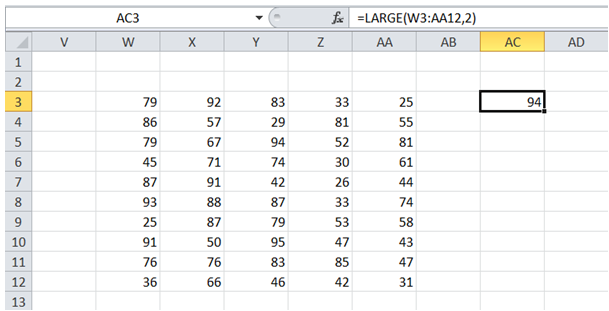## 2nd highest number in range in Excel

To do it in Excel, here is the answer:

a) Enter the formula =LARGE(W3:AA12,2) as shown below where W3:AA12 corresponds to the range that has the numbers.

The second argument 2 corresponds to the position (in this example 2nd highest). This argument could take any value from 1 to the total count of numbers in range.

In case kth lowest number is required, replace "LARGE" in formula above with "SMALL".